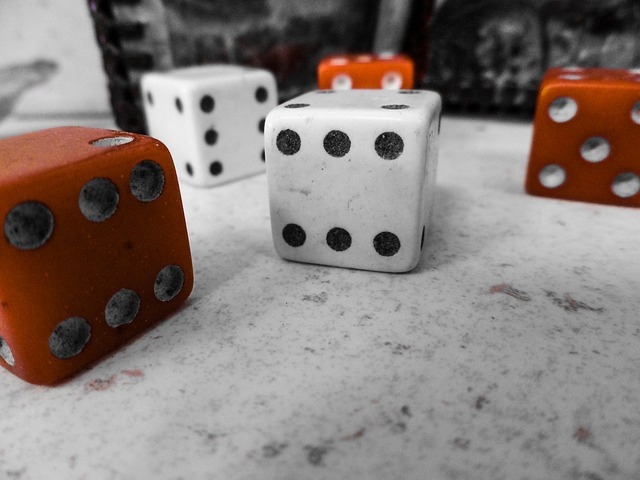Basic Concepts

Learning Outcomes

• Describe a sample space and simple and compound events in it using standard notation
• Calculate the probability of an event using standard notation

Learning new Math vocabulary and notation

You’ve seen before that learning mathematics is similar to learning a new language — it takes repetition and practice to obtain new vocabulary and symbols. Probability is no different. The set of vocabulary and symbols used in probability will likely be completely unfamiliar to you unless you’ve studied probability before. Remember to read the text with your pencil, writing out the terms, definitions, and practice problems multiple times in order to learn them. You’ll need to spend time with these new ways of thinking to make them your own.

If you roll a die, pick a card from deck of playing cards, or randomly select a person and observe their hair color, we are executing an experiment or procedure. In probability, we look at the likelihood of different outcomes.We begin with some terminology.

Events and Outcomes

• The result of an experiment is called an outcome.
• An event is any particular outcome or group of outcomes.
• A simple event is an event that cannot be broken down further
• The sample space is the set of all possible simple events.

example

If we roll a standard 6-sided die, describe the sample space and some simple events.

Basic Probability

Given that all outcomes are equally likely, we can compute the probability of an event E using this formula:

$P(E)=\frac{\text{Number of outcomes corresponding to the event E}}{\text{Total number of equally-likely outcomes}}$

examples

If we roll a 6-sided die, calculate

1. P(rolling a 1)
2. P(rolling a number bigger than 4)

This video describes this example and the previous one in detail.

Let’s say you have a bag with 20 cherries, 14 sweet and 6 sour. If you pick a cherry at random, what is the probability that it will be sweet?

Try It

At some random moment, you look at your clock and note the minutes reading.

a. What is probability the minutes reading is 15?

b. What is the probability the minutes reading is 15 or less?

Cards

A standard deck of 52 playing cards consists of four suits (hearts, spades, diamonds and clubs). Spades and clubs are black while hearts and diamonds are red. Each suit contains 13 cards, each of a different rank: an Ace (which in many games functions as both a low card and a high card), cards numbered 2 through 10, a Jack, a Queen and a King.

example

Compute the probability of randomly drawing one card from a deck and getting an Ace.

This video demonstrates both this example and the previous cherry example on the page.

Certain and Impossible events

• An impossible event has a probability of 0.
• A certain event has a probability of 1.
• The probability of any event must be $0\le P(E)\le 1$

Try It

In the course of this section, if you compute a probability and get an answer that is negative or greater than 1, you have made a mistake and should check your work.